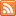# Content Tagged “LO 6.2”## Summary (Unit 3B – Random Variables)

Published: Jan 20th, 2014

Random Variables Binomial Random Variables Continuous Random Variables We have almost reached the end our discussion of probability. We were introduced to the important concept of random variables, which are quantitative variables whose […]

## Did I Get This – Using the Standard Deviation Rule

Published: Feb 8th, 2013

Length (in days) of human pregnancies is a normal random variable (X) with mean 266, standard deviation 16. For the questions below, it would be useful to sketch this normal distribution […]## Normal Random Variables

Published: Feb 8th, 2013

Observations of Normal Distributions The Standard Deviation Rule for Normal Random Variables CO-6: Apply basic concepts of probability, random variation, and commonly used statistical probability distributions. LO 6.2: Apply the standard deviation […]## The “Normal” Shape

Published: Aug 21st, 2012

The Standard Deviation Rule Visual Methods of Assessing Normality Standardized Scores (Z-Scores) CO-4: Distinguish among different measurement scales, choose the appropriate descriptive and inferential statistical methods based on these distinctions, and […]